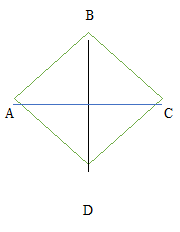# Measurements ( 16 to 20 )

#### Prev     Next

Measurements ( 16 to 20 ):

# APTITUDE QUESTIONS (MEASUREMENTS):

16. The volumes of two cones are in the ratio 1:5 and the radii of the cones are in the ratio of 1:2. What is the length of the wire?

A)    2:5

B)    1:5

C)    3:5

D)    4:5

E)    None of these

Explanation:

The volume of the cone = (1/3)𝜋𝑟2h

Only radius and height are varying so, ignore (1/3)𝜋

V1V2 = r12h1/ r22h2 => 1/5 = (1)2h1/(2)2h2

=> h1/h2 = 4/5

i.e. h1 : h2 = 4:5

17. Find the area of a rhombus whose side is 20 cm and one of the diagonals is 24 cm?

A)    225 sq m

B)    384 sq m

C)    720 sq m

D)    480 sq m

E)    None of these

Explanation:Consider the rhombus ABCD. Let the diagonals intersect at E) since diagonals bisect at right angles in a rhombus.

BE2 + AE2 = AB2

202 = 122 + AE2AE = √(400 − 144) = √256 = 16

AC = 16 + 16 = 32 cm

Area of rhombus = ½ * d1d2

= ½ * 32 * 24 = 384 sq cm

18. The length of a rectangle is two-fourth of the radius of a circle. The radius of the circle is equal to the side of the square, whose area is 1156 sq units. What is the area(in sq units) of the rectangle if the breadth is 10 units?

A)    140

B)    156

C)    175

D)    214

E)    None of these

Explanation:

Given that the area of the square = 1156 sq units

=> Side of square = √1156 = 34 units

=> The radius of the circle = side of the square = 34 units length of the rectangle = 2/4 * 34 = 17 units

=> Given that breadth = 10 units

=> Area of the rectangle = lb = 17 * 10 = 170 sq. units

19. The sector of a circle has a radius of 14 cm and central angle 140°. Find its perimeter.

A)    61.5 cm

B)    63.5 cm

C)    64.5 cm

D)    62.2 cm

E)    None of these

Explanation:

Perimeter of the sector = length of the arc + 2(radius)

= (140/360 * 2 * 22/7 * 14) + 2(14)

= 34.2 + 28 = 62.2 cm

20. The difference between the length and breadth of a rectangle is 13m. If its perimeter is 106m, then its area is:

A)    660 sq.m

B)    420 sq m

C)    480 sq m

D)    520 sq m

E)    None of these

Explanation:

We have (l – b) = 13 and 2(l + b) = 106 or (l + b) = 53

From these two equations we get l = 33 and b = 20

∴ area = (l * b) = (33 * 20) m2 = 660 m2